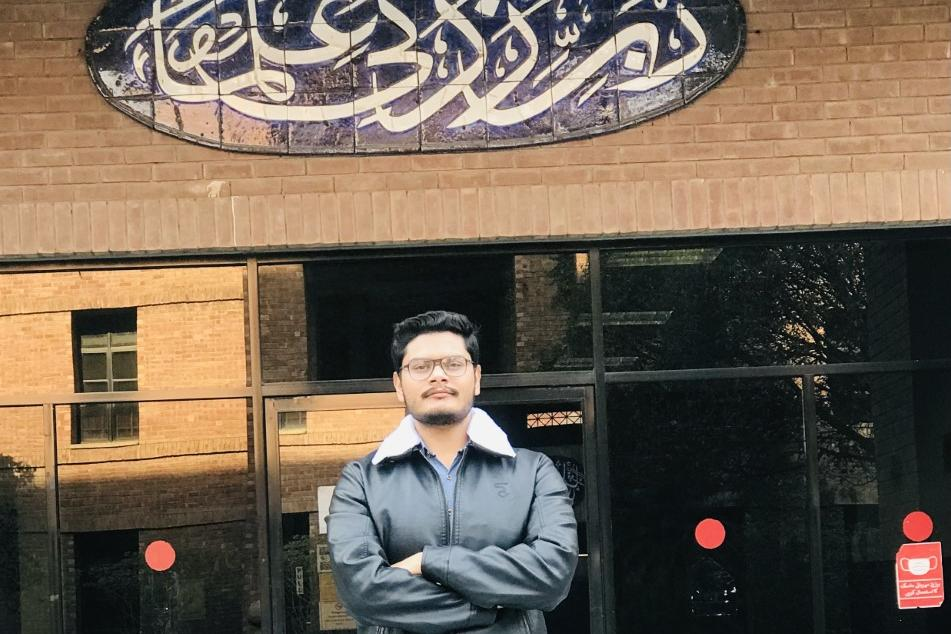Event date:
May 21 2021 12:30 pm

## Hamiltonian Operators and First Integrals for System of Ordinary Differential Equations

Supervisor
Dr. Imran Naeem
Student
Zartab Ali
Venue
Zoom Meetings (Online)
Event
MS Thesis defense
Abstract
The notion of Lagrangian, partial Lagrangian, Hamiltonian and partial Hamiltonian are used to obtain closed-form solutions and reduction of ordinary differential equations (ODEs) via first integrals. The Lagrangian and Hamiltonian approaches are algorithmic and systematic to compute the first integrals which can be used for exact solutions of differential equations arise in different areas of sciences, engineering, economics, finance, business etc. In this work, we utilize these techniques to numerous physical models to obtain closed form solutions which provides novel ideas to develop a deep insight and room for analysis of models under considerations. The canonical Hamiltonian systems arise in almost all fields except the economic growth theory and in economic models where current value Hamiltonian system exist. For a state constrained optimal control problem, Pontryagin’s maximum principle provide necessary optimality conditions. Due to state constraints, the resulting system of equations arise from necessary conditions are difficult to solve. We investigate a two-compartment model based on differential equations which is useful for the best treatment for anti-angiogenesis therapy transmission. In this thesis our focus is to classify the arbitrary functions involved in optimization models as well as to study the associated system of ordinary differential equations. The linearization of differential equations will be discussed and we shall show that how one can linearize the non-linear dynamical systems to linear systems.

Meeting ID: 917 3499 3568

Passcode: 122258